Question

Determine the volume of a solid by integrating a cross-section with a triangle Question The solid...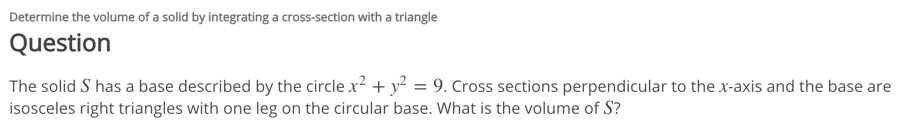Determine the volume of a solid by integrating a cross-section with a triangle Question The solid S has a base described by the circle x' + y2 = 9. Cross sections perpendicular to the x-axis and the base are isosceles right triangles with one leg on the circular base. What is the volume of S?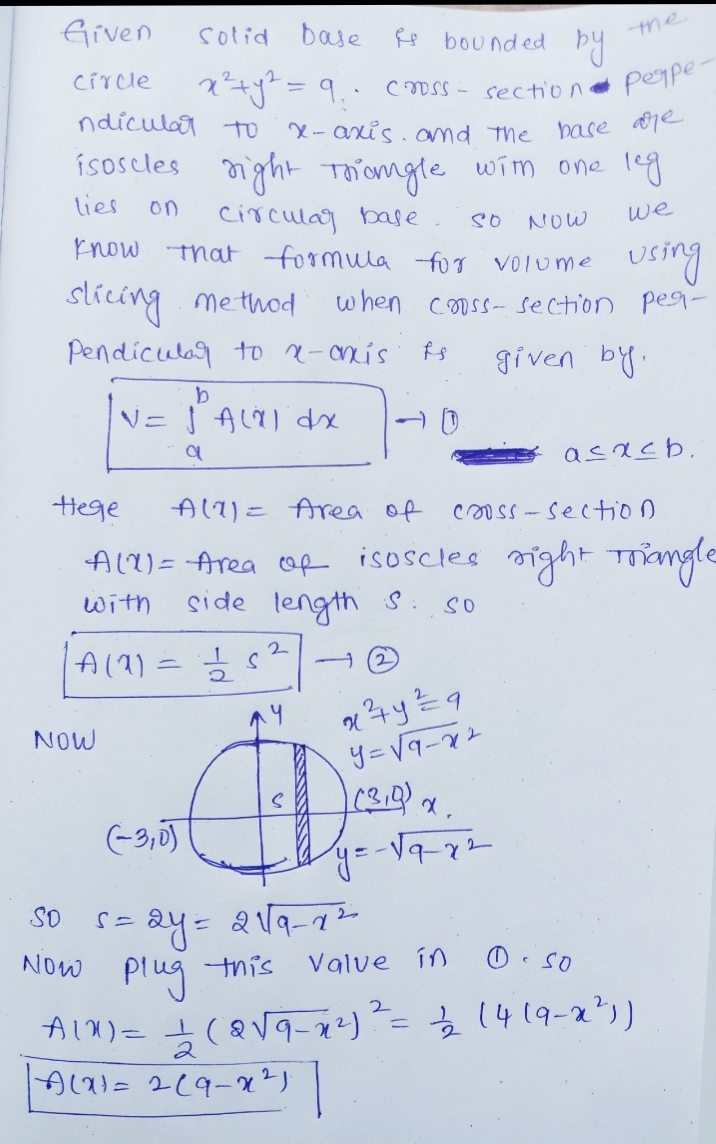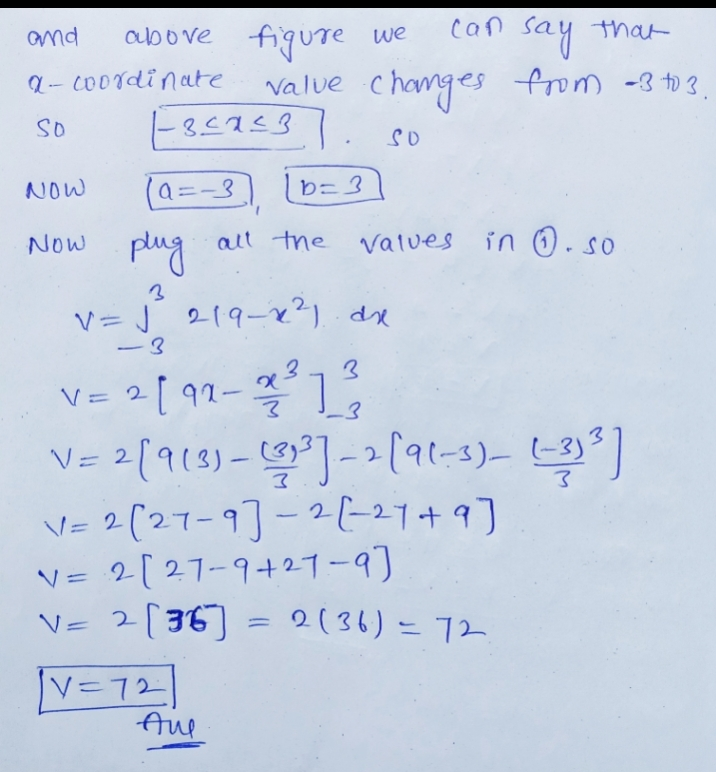Earn Coins

Coins can be redeemed for fabulous gifts.

Similar Homework Help Questions
• Determine the Volume of a Solid by Integrating a Cross-Section With a Circle or Semicircle Question...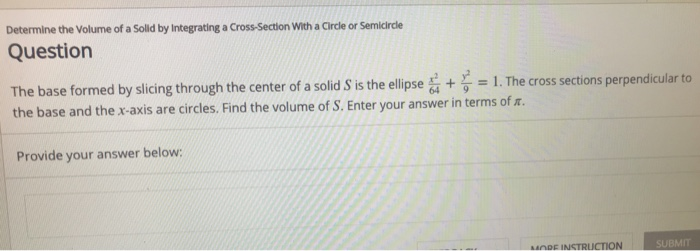Determine the Volume of a Solid by Integrating a Cross-Section With a Circle or Semicircle Question The base formed by slicing through the center of a solid S is the ellipse + y,-1. The cross sections pe the base and the x-axis are circles. Find the volume of S. Enter your answer in terms of r. 64 9 Provide your answer below: MODE INSTRUCTION MI

• Find the volume of the solid via cross-sections?

The base of a solid is a circular disk with radius 3. Find the volume of the solid if parallel cross-sections perpendicular to the base are isosceles right triangles with hypotenuse lying along the base. Answer key: #V=36#

• find the volume of the solid

Find the volume of the solid:The base of S is an elliptical region with boundary curve 9x2 + 16y2 = 144. Cross-sections perpendicular to the x-axis are isosceles right triangles with hypotenuse inthe base.

• 11. Find the volume of the given right tetrahedron. (Hint: Consider slices perpendicular to one of...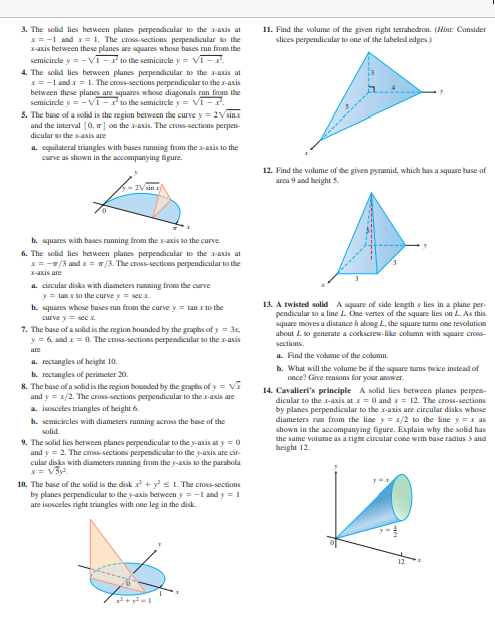11. Find the volume of the given right tetrahedron. (Hint: Consider slices perpendicular to one of the labeled edges.) 3. The solid lies between planes perpendicular to the x-axis at x= -1 and x = 1. The cross-sections perpendicular to the I-axis between these planes are squares whose bases run from the semicircle y = -VI-to the semicircle y = VI- 4. The solid lies between planes perpendicular to the x-axis at x= -1 and .x = 1. The cross-sections...

• The last one was incorrect Use the general slicing method to find the volume of the...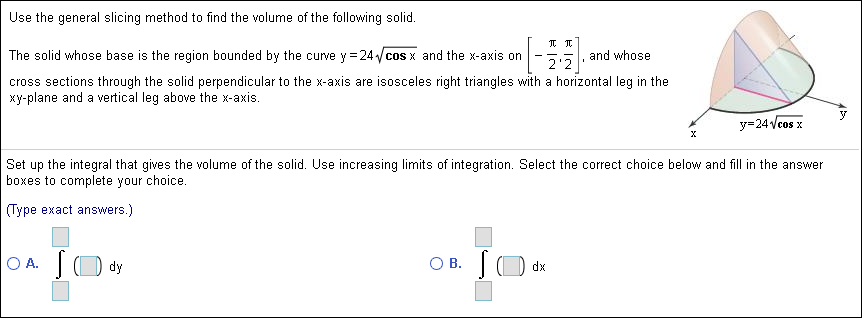The last one was incorrect Use the general slicing method to find the volume of the following solid. The solid whose base is the region bounded by the curve y 24cos x and the x-axis on and whose 2'2 cross sections through the solid perpendicular to the x-axis are isosceles right triangles with a horizontal leg in the xy-plane and a vertical leg above the x-axis. y 24Vcos x Set up the integral that gives the volume of the solid....

• Find the volume of the solid generated by revolving the region R bounded by the graphs...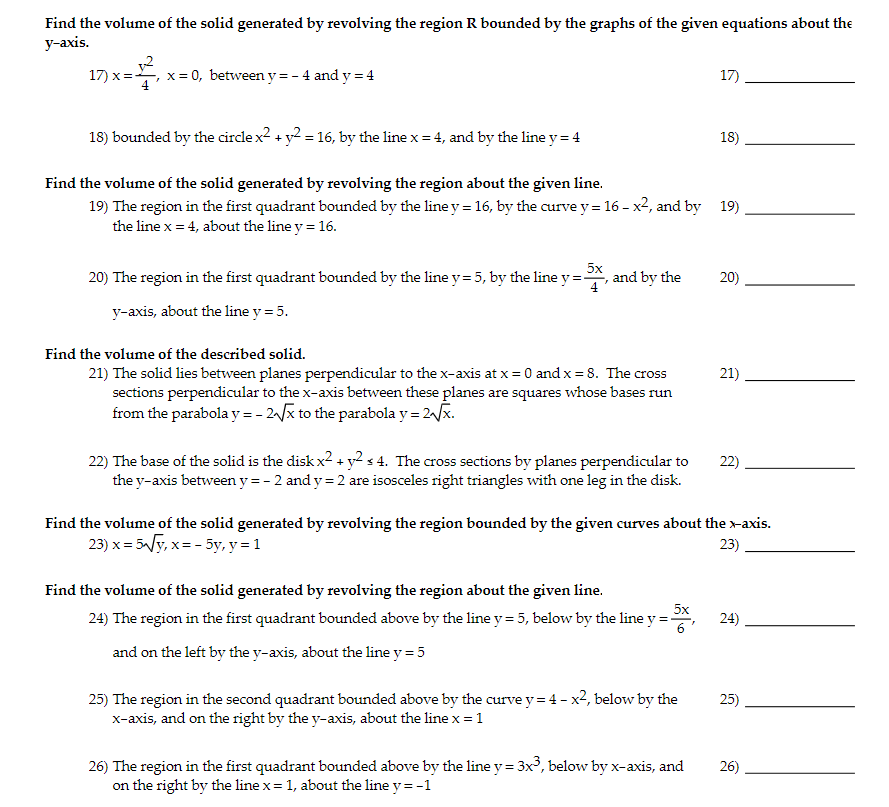Find the volume of the solid generated by revolving the region R bounded by the graphs of the given equations about the y-axis. 17)x= x=0, between y=- 4 and y = 4 17) 18) bounded by the circle x2 + y2 = 16, by the line x = 4, and by the line y = 4 18) Find the volume of the solid generated by revolving the region about the given line. 19) The region in the first quadrant bounded...

• 1. Given a solid whose base is a circle of radius 5 inches and each cross-section...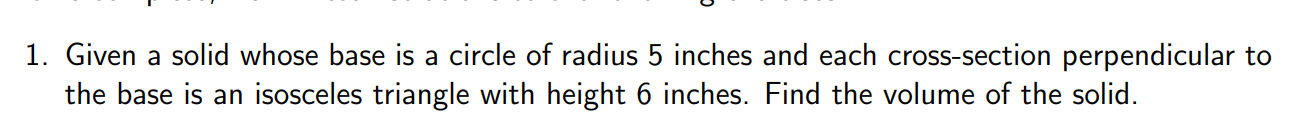1. Given a solid whose base is a circle of radius 5 inches and each cross-section perpendicular to the base is an isosceles triangle with height 6 inches. Find the volume of the solid.

• Let the region bounded by x^2 + y^2 = 9 be the base of a solid. Find the volume if cross sections taken perpendicular to the base are isosceles right triangles. A). 30 B). 32 C). 34 D). 36 E). 38

Let the region bounded by x^2 + y^2 = 9 be the base of a solid. Find the volume if cross sections taken perpendicular to the base are isosceles right triangles. A). 30 B). 32 C). 34 D). 36 E). 38

• A volume is described as follows: 1. the base is the region bounded by x y2 + 6y + 109 and x-y2-26y + 187; 2. every cross section perpendicular to the y-axis is a semi-circle. Find the volume of this...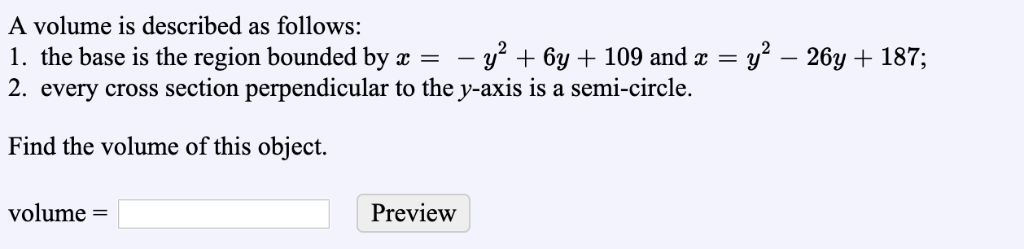A volume is described as follows: 1. the base is the region bounded by x y2 + 6y + 109 and x-y2-26y + 187; 2. every cross section perpendicular to the y-axis is a semi-circle. Find the volume of this object. Preview volune A volume is described as follows: 1. the base is the region bounded by x y2 + 6y + 109 and x-y2-26y + 187; 2. every cross section perpendicular to the y-axis is a semi-circle. Find the...

• 5) The following integrals compute the volume of a solid with a known cross-section. For each...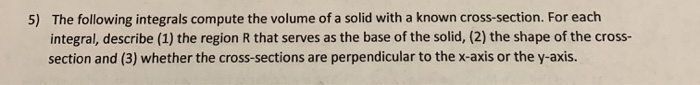5) The following integrals compute the volume of a solid with a known cross-section. For each integral, describe (1) the region R that serves as the base of the solid, (2) the shape of the cross- section and (3) whether the cross-sections are perpendicular to the x-axis or the y-axis. (c) (Iny)? dy